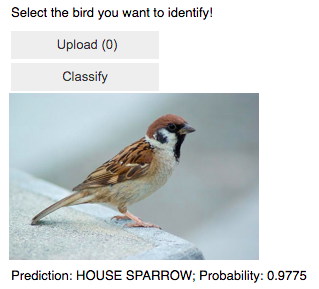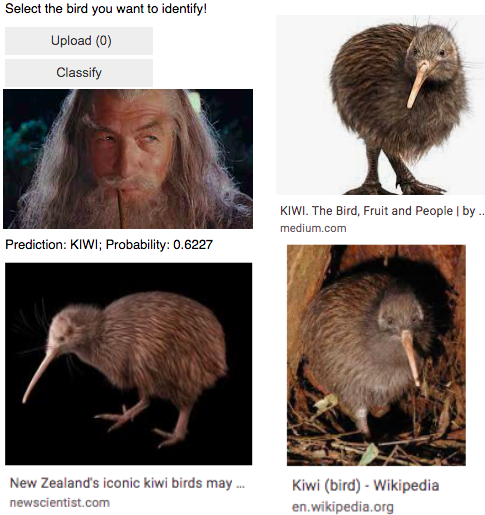计算机视觉技术在Kaggle数据集上的应用• 这里是来自Fast.ai库的一个中等大小的玩具数据集，
• 这里是来自Fast.ai库的大型玩具数据集，
• 在此导出外部数据集，
• 在这里通过网络搜索创建我们自己的数据集。

A.如何在Colab中使用Kaggle数据集，以及

B.如何使用Fast.ai快速手册的第07课中介绍的归一化、调整大小和测试时间放大计算机视觉技术。Lesson 07 in the Fast.ai FastBook

A.在Colab中使用Kaggle数据集

a.如果你还没有™账户，那就创建一个。它是免费的，而且处理得很快。

b.探索您感兴趣的数据集/比赛。这个博客涉及鸟类的计算机视觉/图像分类。computer vision/ images classification for birds

c.为每个类选择至少包含20幅图像的数据集。否则，您可能会遇到错误。

d.我已经能够对图像使用.jpg和.png格式。如果遇到.tfrec格式，请查找指向原始数据集的链接。

e.在安装前完成此操作。如果您进行了设置并分心，笔记本运行可能会断开连接，您需要重新运行。

2.设置。

a.笔记本

b.安装和进口

i.Fast.ai库的一般设置。

!pip install -Uqq fastbook
import fastbook
fastbook.setup_book()
from fastbook import *
#!pip install fastai -U # unhash if this is your first use
import fastai
from fastai.vision.all import *

• 在kaggle页面中，打开您的“EUROURE˜”帐户“EUROU™”。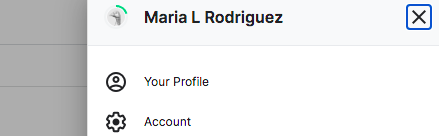• 创建新的API令牌。• 在Colab笔记本中，安装Kaggle并上传API Token/Kaggle json文件。

!pip install -q kaggle

• 创建Kaggle目录并启用访问。

!mkdir ~/.kaggle
!cp kaggle.json ~/.kaggle/

3.收集您的数据。

a.下载代码格式。

• ！卡格尔
• 数据集还是竞赛集(例如，在Kaggle中，您的集合是来自数据集还是竞赛部分？)
• 下载
• 路径

b.从下载的压缩文件中获取单个文件，并在完成后删除该压缩文件。

!unzip \*zip && rm *.zip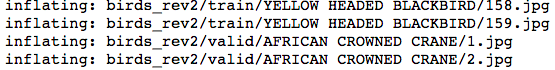c.指定路径。

train_path = 'birds_rev2/train'

That-Euro™就是这样！不需要创建数据帧或进行任何其他预处理。

B.高级成像技术示例。

dblock = DataBlock(
(ImageBlock(), CategoryBlock()),
get_items = get_image_files,
get_y = parent_label,
splitter = RandomSplitter(seed=42),
item_tfms = Resize(460),
batch_tfms = aug_transforms(size=224))
dls.train.show_batch()dls.train.show_batch(unique=True)model = xresnet50(n_out = dls.c)
learn_base = Learner(dls, model, loss_func = CrossEntropyLossFlat(),
metrics = accuracy)
learn_base.fit_one_cycle(5, 3e-3)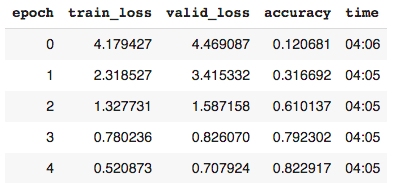• Learner是Fast.ai中的代码类，用于组装数据、模型和培训。
• Xresnet是一种顺序的、非预先训练的神经网络。
• n_out=dls.c表示类别或标签的数量。
• 交叉熵损失是模型能够学习的计算。它是从类的预测概率导出的。概率值的范围从0到1。当这些值被转换为交叉熵时，接近0(非常差的预测)的概率变得更加明显，因此受到的惩罚更多。
• 交叉熵损失平坦是交叉熵损失的重组，便于处理。

learn_base.lr_find()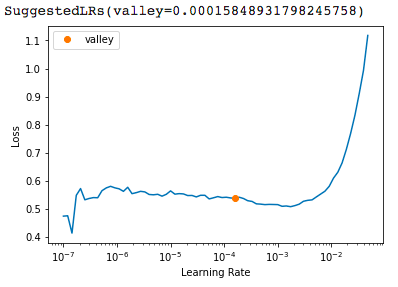learn_base.show_results()2.应用归一化变换。

dblock = DataBlock(
(ImageBlock, CategoryBlock),
get_items = get_image_files,
get_y = parent_label,
item_tfms = Resize(460),
batch_tfms = [*aug_transforms(size=224),
Normalize.from_stats(*imagenet_stats)]) #

x, y = dls.one_batch()          # baseline
xn, yn = dls_norm.one_batch() # normalized

print('Non-normalized tensors:', x[:10])
print('Mean:',x.mean(dim = [0,2,3]))
print('Std:', x.std(dim = [0,2,3]))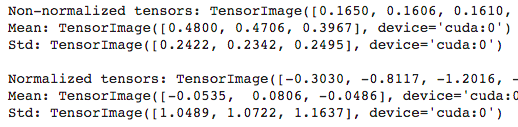model = xresnet50(n_out = dls_norm.c)
learn_norm = Learner(dls_norm, model, loss_func = CrossEntropyLossFlat(), metrics = accuracy)
learn_norm.fit_one_cycle(5, 3e-3)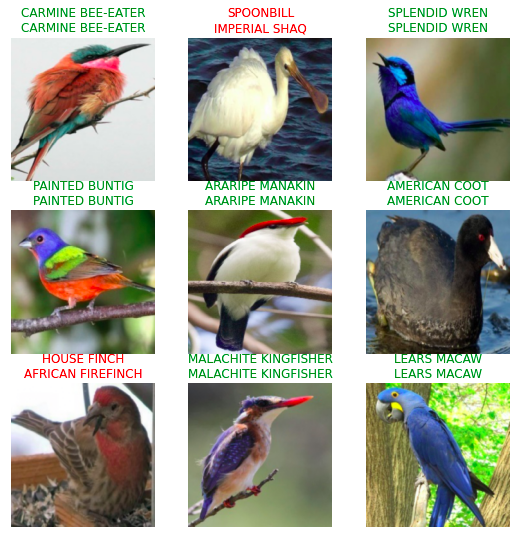3.应用渐进式大小调整

dblock = DataBlock(
(ImageBlock, CategoryBlock),
get_items = get_image_files,
get_y = parent_label,
item_tfms = Resize(460),
batch_tfms = aug_transforms(size=128)) # start small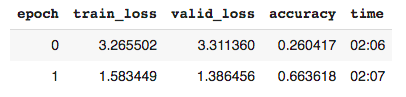dblock = DataBlock(
(ImageBlock, CategoryBlock),
get_items = get_image_files,
get_y = parent_label,
item_tfms = Resize(460),
batch_tfms = aug_transforms(size=224)) # bigger

learn_128.dls = dls_224
learn_128.fit_one_cycle(3, 3e-3)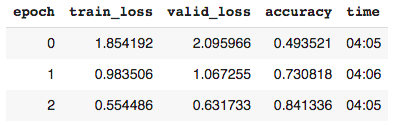4.使用测试时间增加(TTA)。

# using the baseline dblock and dls
model = xresnet50(n_out = dls.c)
learn = Learner(dls, model,
loss_func = CrossEntropyLossFlat(),
metrics = accuracy)

5.结合这些先进的转换技术。

dblock = DataBlock(
(ImageBlock, CategoryBlock),
get_items = get_image_files,
get_y = parent_label,
item_tfms = Resize(460),
batch_tfms = aug_transforms(size=128)) #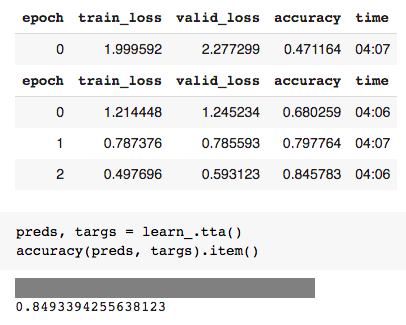interp = ClassificationInterpretation.from_learner(learn_)
interp.most_confused(min_val = 5)6.让？Euro™‘s发挥吧！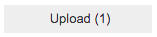out_pl = widgets.Output()
out_pl.clear_output()
with out_pl: display(img.to_thumb(250))
pred, pred_idx, probs = learn_.predict(img) # rev
lbl_pred = widgets.Label()
lbl_pred.value = f'Prediction: {pred}; Probability: {probs[pred_idx]:.04f}'
btn_run = widgets.Button(description = 'Classify')
btn_run